Testing of Gender Differences on Sib-Sib Correlations for Binary Traits: Likelihood Based Inference with Application to Arterial Blood Pressures Data
Journal of Biometrics & Biostatistics

Like us on:

# Testing of Gender Differences on Sib-Sib Correlations for Binary Traits: Likelihood Based Inference with Application to Arterial Blood Pressures Data

Mohamed Shoukri1,3*, K Collison2,3 and F Al-Mohanna1,2,3

1National Biotechnology Center, King Faisal Specialist Hospital and Research Center, Riyadh, Saudi Arabia

2Department of Cell Biology, King Faisal Specialist Hospital and Research Center, Riyadh, Saudi Arabia

3College of Medicine Al-Faisal University, Riyadh, Saudi Arabia

*Corresponding Author:
Mohamed Shoukri
National Biotechnology Center-King Faisal Specialist Hospital and Research Center
Tel: +966-509491454
E-mail: [email protected]

Received date: January 16, 2014; Accepted date: February 22, 2014; Published date: February 28, 2014

Citation: Shoukri M, Collison K, Al-Mohanna F (2014) Testing of Gender Differences on Sib-Sib Correlations for Binary Traits: Likelihood Based Inference with Application to Arterial Blood Pressures Data. J Biomet Biostat 5:186. doi: 10.4172/2155-6180.1000186

Copyright: © 2014 Shoukri M, et al. This is an open-access article distributed under the terms of the Creative Commons Attribution License, which permits unrestricted use, distribution, and reproduction in any medium, provided the original author and source are are credited.

Visit for more related articles at Journal of Biometrics & Biostatistics

#### Abstract

Estimation of measures of familial aggregation is considered the first step in establishing whether a specified disease has a genetic component. Population based family study designs areusually used to estimate correlations among siblings. When the trait of interest is quantitative (e.g. blood pressure, body mass index, blood glucose level) testing the effect of gender differences on sib-sib correlations is achieved using the likelihood method of estimation under the assumption of multivariate normality. When the trait of interest is measured on the binary scale testing the equality of a brother-brother and sister-sister correlation is more complex. In this paper we develop likelihood-based inference procedures for this purpose which may beapplied to nuclear family data.

#### Keywords

Family studies; Multivariate beta-binomial distribution; Likelihood estimation; Score test

#### Introduction

Population based family studies have been used in genetic epidemiology to assess the association of environmental risk factors with disease and to quantify the aggregation of cases within families. These types of studies integrate statistical methods and classical epidemiology to analyze the correlations among family members who share the same genetic and environmental background. There are several advantages in using family study designs. The use of extended pedigrees or even nuclear families enhances the statistical power for gene discovery. Clinical characteristics common to family members may also be used to increase information by defining subgroups of families for analysis such as in the investigation of familial aggregation of components of metabolic syndrome . Another important feature of family studies in contrast to studies of unrelated individuals is the issue of internal control. The analysis of traits of interest for family members should account for both genetic background factors and environmental exposures. A common example is the case of monozygotic twins where maximum genetic control is achieved. It is well-known that nuclear family members tend to have relatively similar environmental conditions, diet, and perhaps levels of physical activity. The familial aggregation of much chronic and infectious disease is also well documented. For example, results from recent studies have shown that pathogens causing Th1 diseases are passed from parents to child. Information accessed on December 23-2012 from (http:// bacteriality.com/2008/07/31/hpv/) reveals that some of the chronic bacterial species that cause inflammatory illness can remain alive in breast milk and thus be passed from mother to child through breast feeding. Growing evidence suggests that the Th1 pathogens, rather than genetic mutation, are the driving force behind this familial aggregation. Although their role is unclear, researchers have also found a relationship between bacterial infection and cancer . This chain of reasoning provides a possible explanation for the aggregation of cancer in families. A study conducted in 2010 using the PET scanner to examine the prevalence of plaque in brains (which is the hallmark ofAlzheimer’s disease) found that a child’s level of plaque is consistent with the corresponding levels of their fathers and in particular of their mothers, even years before the child’s diagnosis .

In many population-based family studies, interest is focused in detecting gender differences in the risk of developing a chronic disease. For example, a recent study  aiming at examining sex-specific associations between cardiovascular risk factors and type 2 diabetes mellitus showed that there are gender-related dissimilarities that are apparently involved in disease development. Another study conducted on a sample of families from South Australia  found that men and women face different challenges in the management of diabetes and its associated complications.

One of the major limitations of the above studies is that the comparisons between males and females were based on parallel group designs, and consequently suffer from the lack of control over possible confounding. Another limitation is that the lack of a reference population makes the problem of statistical inference (estimation and hypothesis testing) less meaningful. A further methodological challenge that faces researchers is that estimates of trait correlations, specifically the intra-class correlations for males and females, are themselves correlated. Although studies on comparing sib-sib correlations have been of frequent interest [6,7] comparisons among these correlations have been usually made descriptively. When traits are measured on the continuous scale, Donner et al.  developed several procedures for comparing the sib-sib correlations among males and females, including likelihood-based tests, while assuming that the underlying mechanism generating the data is multivariate normal. When the trait ofinterest is measured the binary scale, efficient methods for comparing sib-sib correlations characterizing males and female have not yet been developed.

This paper has a threefold objective. First, we develop a multivariate probability distribution for the vector of binary observations based on a random sample of independent sib-ships. The vector will be split into two sub-clusters, separating female responses from male responses. Second we construct the likelihood function of the sample as based on the joint distribution of the created sub-clusters. This allows us to develop score and Wald chi-square-tests of significance that compare the levels of similarity among males and females from the same family. Finally, we illustrate our procedures using published arterial blood pressures data.

#### Models

Suppose that we have a random sample of k sib-ships, where each sib-ship constitutes a cluster. Let yi=(yi1, yi2,…, , xi1, xi2,… )T denote the vector of observations from the ith cluster, where bi=number of brothers in the ith family, si=number of sisters in the ith family, ni=bi+si=sibship size of the ith family,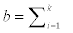bi= number of brothers in the sample of k families,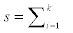si=number of sisters in the sample of k families, and N=b+s= number of siblings in the k families. It is clear then that each cluster (sib-ship) is naturally divided into two sub-clusters, one cluster represents brothers and the other sub-cluster represents sisters.

Let yij=1(0) denote the presence (absence) of a trait observed on the jth brother from the ith family (j=1,2,…bi;i=1,2,…k). Similarly, let xij=1(0) denote the presence (absence) of this trait as observed on the jth sister in the ith family (j=1,2,…si;i=1,2,…k). Let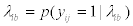denote the probability that a randomly selected brother from the ith family is classified as having the trait of interest, and let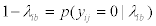Moreover let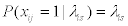and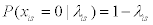. We initially assume that the distribution of the brothers’ scores is conditionally independent of the distribution of the sisters’ scores. To introduce the correlation among brothers within the ith family we shall assume that λib is an element of a random sample obtained from a beta distribution with parameters (αb,βb) so that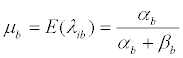,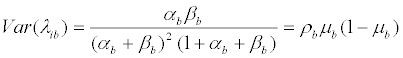Where ρb=(1+αbb)-1.

Similarly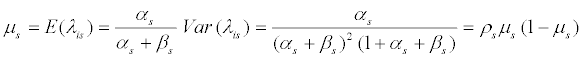,

where ρs=(1+αss)-1.

We can show that the population common intraclass correlation among brothers in the samesub-cluster is:

Corr (yij, yij ׳)=ρb,

and the common intraclass correlation among sisters in the other subcluster is:

Corr (xim, xil ׳)=ρs

i≠j ׳ =a,2,…bi, and m≠l=1,2,…si for all i=1,2,…k.

We further define the interclass correlation among brothers and sisters as:

Corr (yij, xil)=ρ12 i=1,2,…k, j=1,2,…bi and l = 1,2,…si.

Note that, because of the exchangeability condition, the unconditional distribution of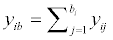is that of a betabinomial distribution with:

E(yib)=biμb            (1)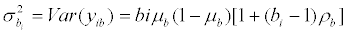(2)

Similarly, the unconditional distribution of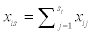will be that of a beta-binomial distribution with

E(xis)=siμs            (3)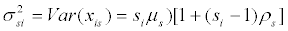(4)

Details may be found in references [9-11].

The beta- binomial probability distributions of yib and xis are given respectively as: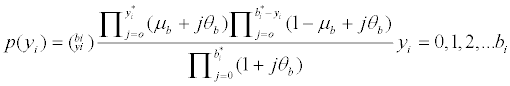(5)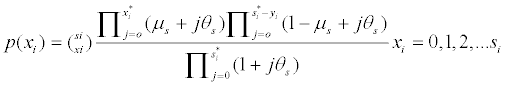(6)

(α*=a-1).ϑbb/(1-ρb), with a similar transformation for ϑs=ρs/(1-ρs).

The above set-up assumes that the three sibling correlations ρb, ρs, and ρbs are constant among families in the parent population. With this assumption our main interest is in developing a likelihood-based approach for testing several hypotheses that are inherently related. We first construct a bivariate distribution based on the marginal distributions given in (5) and (6) whichincludes all the parameters of interest. However a different approach is needed to construct thebivariate distribution of the sibling scores characterized by the interclass correlation. This approach, developed by Sarmanov  and Lancaster , is known as Positive Dependence byExpansion (PDE). Danaher  proposed a simplified and flexible form of the distribution basedon Lancaster’s representation.

We are interested in testing the following hypotheses:

1- H0 : ρ12=0

2- H0 : ρbs

As a first step, we follow Lancaster  in constructing a bivariate distribution by joining the marginal distributions given in (5) and (6). The resulting representation is given by: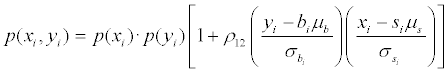(7)

Yi=0,1,2,...bi, and xi=0,1,2,… si. Direct computations show that Corr(xi,yi)=ρ12. The sum of theright hand-side of equation (7) over all the possible values of (xi,yi) is one. Therefore, the equationrepresents a proper bivariate probability distribution with parameters vector φ=(μbsbs12)'.

#### Methods

Our inferences on the parameters of interest are based on the likelihood principle. To test the hypothesis H012=0 against the alternative H112>0, we assume that a random sample of k sib-ships is available. The log- likelihood function of the sample is given by: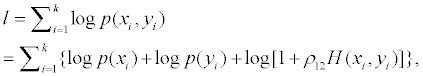Where,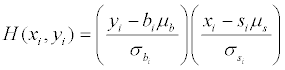. The score function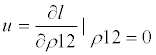is given by: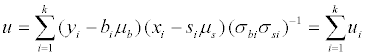By the central limit theorem and for fixed bi and si, the distribution of each component ui tends uniformly to the standard normal distribution under H0 as k →∞ . Moreover, we can show that under H0 that the statistic s2=u2/k will be asymptotically distributed as chisquare with one degree of freedom . This statistic is the locally most powerful one-sided test of H012=0 against H112>0. Full details of the proof can be found [15,16]. Moran  showed that if the remaining parameters are replaced by any consistent estimators under the null hypothesis the asymptotic properties of this test statistic will be preserved. Such consistent estimators can be either the maximum likelihood (MLE) or the moment estimators.

In Table 1 we provide estimates of the sample sizes (number of sib-sips) needed to detect thedeparture from H012=0 in the direction of a two sided alternative under several scenarios. We limited our computations to the balanced case with equal response rates. It can be seen from Table 1 that when we have a small departure from the null hypothesis, a large sample is needed, regardless of the sib-ship sizes. Moreover, when the response rates are far from their boundary values (0, 1), a substantially smaller number of sib-ships are needed. Tables 2 and 3 present the empirical powers, when the design isbalanced (number of brothers equals number of sisters within the same family), for μbs=0.1 and μbs=0.5, respectively. It is clear that the power increases with the increase in the number of sib-ships, and is unaffected by sib-ship sizes. Again, a noticeable increase in the power is achieved when the response rates are far from their boundary values. In Table 4 we show that the effect of unbalanced design (number of brothers does not equal number of sisters within the same family) on the power is nottangible.

 µb=µs=.1 µb=µs=.5 ρb ρs ρ12 b=s 2 5 10 b=s 2 5 10 .2 .2 .1 715 697 693 612 612 612 .2 .5 .1 715 613 613 612 612 612 .5 .5 .5 44 44 44 22 22 22 .8 .8 .5 45 41 41 22 22 22 .8 .8 .8 21 21 21 7 7 7

Table 1: Sample size requirements for Type I error rate 5% and power 80%. To test the hypothesis H012=0.

 µb=µs=.1 k=25 k=50 k=100 ρb ρs ρ12 b=s 2 5 10 b=s 2 5 10 b=s 2 5 10 0 0 0 .051 .051 .051 .051 .051 .051 .051 .051 .051 .2 .2 0 .051 .051 .051 .051 .051 .051 .051 .051 .051 .5 .5 .2 .336 .334 .334 .440 .440 .440 .594 .595 .595 .8 .8 .5 .661 .661 .660 .820 .820 .820 .950 .950 .950 .8 .8 .8 .830 .830 .830 .950 .950 .950 .990 .990 .990

Table 2: Power Calculations for testing H012=0.

 µb=µs=.5 k=25 k=50 k=100 ρb ρs ρ12 b=s 2 5 10 b=s 2 5 10 b=s 2 5 10 0 0 0 .051 .051 .051 .051 .051 .051 .051 .051 .051 .2 .2 0 .051 .051 .051 .051 .051 .051 .051 .051 .051 .5 .5 .2 .336 .334 .334 .489 .489 .489 .643 .643 .643 .8 .8 .5 .661 .661 .660 .820 .820 .820 .950 .950 .950 .8 .8 .8 .839 .839 .839 .985 .985 .985 .999 .999 .999

Table 3: Power Calculations for testing H012=0.

ρb ρs ρ12 b s k=25 k-50 k-100
.5 .5 .2 2 4 .257 .409 .643
.5 .5 .2 10 5 .258 .411 .645
.8 .8 .5 10 2 .841 .986 .999
.8 .8 .5 6 3 .840 .985 .999
.2 .2 .2 2 4 .260 .409 .650

Table 4: Power calculations for testing H012=0, µbs=.5, Under unbalanced design.

#### Maximum Likelihood Estimation

The MLE’s of the model parameters are obtained by simultaneously solving the likelihood equations: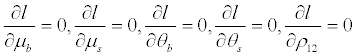We obtain the variance-covariance matrix Σ of the MLE’s by inverting the matrix of the negative of the second partial derivatives of ℓ with respect to the five parameters. The two matrices are given as: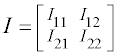, and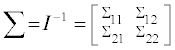.

Here, Σ is the variance-covariance matrix of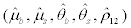. To find the elements of Σ we use the method of matrix partitioning .

The matrix I11 is a 2×2 and symmetric, I12=I'21 is a 2×3 matrix and I22 is a 3×3 diagonal matrix. The elements of the covariance matrix are given in closed form as: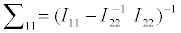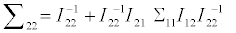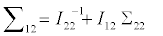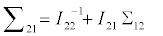In the Appendix we provide expressions for the elements of I and Σ.

Hypothesis testing

In this section we develop an approach for testing the effect of gender differences on sib-sib correlations. If the trait of interest is normally distributed, the sib means and the sib-sib correlations are orthogonal to each other, implying the expected value of the second partial derivatives of the likelihood function withrespect to the mean and correlation is zero . This orthogonality also implies that the maximum likelihood estimators of these parameters are asymptotically independent. Therefore, as in Donner et al.  we can test the equality of ρb and ρs independent of the values of μb and μs. For the bivariate beta-binomial distribution, the orthogonality condition is not satisfied and therefore we propose anomnibus test in the form: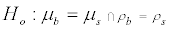(8)

This hypothesis takes into account the correlations among all the estimated parameters. Let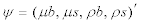and consider the affine transformation.

H0:Aψ=0 versus H1:Aψ=δ>0

The matrix A has 2 rows and 4 columns and is specified as: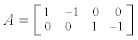To test the stated hypothesis, an omnibus test statistic is constructed, using the asymptotic distributional properties of the MLE of ψ. From Serfling , the MLE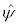has the asymptotic distribution: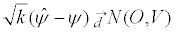where V is the variance-covariance matrix ofand is obtained by deleting the 5th row and the 5th column of Σ. Letting H=Aψ, the question reduces to testing H0: H=δ>0.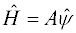is therefore distributed as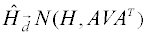.

Hence, from Graybill  the quadratic form: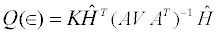(9)

is asymptotically non-central chi-square with 2 degrees of freedom and non-centrality parameter

є=KHT(AV AT)-1 H

Moreover, є=0 if and only if H0 is true. Hence referring Q(0) to the table of chi-square distribution with 2 degrees of freedom, H0: H=0 is rejected if Q(0) exceeds the tabulated value of a chi-square with 2 degrees of freedom at the chosen level of significance.

Example: Mial and Oldham’s blood pressure data

The data used for illustration here are obtained from a survey that aimed at assessing the levels of similarity in systolic and diastolic blood pressure among family members living within 25 miles of Rhonda Fach Valley in South Wales and published by Miall and Oldham  previously analyzed [23,24]. Observations were made on parents and their offspring, with each observation consisting of systolic and diastolic blood pressures measured to the nearest 5 mmHg. However among 250 sampled families, only 204 contained information on brothers and sisters. Furthermore, because of the impossibly low systolic blood pressure (15 mmHg) for one daughter, another family was omitted leaving 203 families for the analysis. Since these data weregiven on a continuous scale, we dichotomized the observations such that for an individual whose systolic/diastolic blood levels above 130/80, the assigned binary score was 1, otherwise, set as 0. The results of the data analysis are summarized in Tables 5 and 6. Table 5 shows the maximum likelihood of the model parameters, together with their standard errors. Table 6 displays the variances and covariances among the estimated parameters using the expression in the Appendix.

Parameter Estimate ± SE
µb .294 ± 0.028
ρb .200 ± 0.077
µs .217 ± 0.026
ρs .274 ± 0.071
ρ12 .195 ± .095

Table 5: Estimates of the model of parameters ± standard error.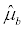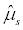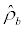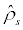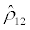.8×10-3 -.15×10-3 .1×10-3 0 .19×10-3.7×10-3 0 .1×10-3 .13×10-3.6×10-2 .2×10-3 .83×10-3.5×10-2 .88×10-3.90×10-2

Table 6: Variance-Covariance matrix of the estimates.

The null hypothesis H0: ρ12=0 tested against the one-sided alternative H1: ρ12>0 is rejected as s2=4.48 (p-value=.034). The Wald one degree of freedom chi-square test w=(0.195/0.095)2=4.21, leading to the same conclusion as the score test. To test the null hypothesis (8) we use the statistic givenin (9). Direct computation shows that Q(0)=3.246, (p=0.197). Therefore, we conclude that there is no sufficient evidence to support the claim of gender differences in the distribution of hypertension basedon this data set. An equally important hypothesis to be tested is whether gender has influence on the dependence structure. That is we need to test whether within gender correlations are the same as across gender correlation. This hypothesis can be easily formulated as:

H0bs12. We may then formulate the simple contrast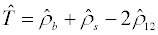Under the null hypothesis, T is asymptotically unbiased, that is E(T)=0, and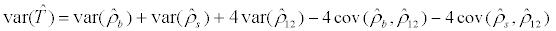. Therefore, asymptotically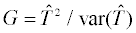has a chi-square distribution with one-degree of freedom. From the data, G=0.565, and a p-value=0.452. Therefore, based on this data we conclude that the correlations within gender are the same across genders.

#### Remarks

Originally the Miall and Oldham’s data are measured on the continuous scale. Under the assumption of multivariate normality Mian and Shoukri  used the MLE to produce the following estimates for the within gender and across gender correlations. We summarize the results in Table 7. For testing H0bs, a one degree of freedom chi-square test statistic is 61, with p-value<0.00001. Similar to the above approach, H012=0 is rejected (p-value<0.00001). Similarly H0bs12 has a chi-square value=26.48, with p-value<0.00001.

Parameter Systolic Diastolic
ρb 0.146 ± 0.073  0.163 ± .073
ρs 0.32 ± 0.069 0.248 ± .070
ρ12 0.178 ± 0.054 0.215± .052

Table 7: Estimates of Correlation parameters for original data.

It is clear that the dichotomization resulted in a reduction in the efficiency of the maximum likelihood estimates of the sibling correlations, which would result in a substantial loss of power of detecting departure from the null hypotheses of interest. This issue has been a subject of discussion by many authors [25,26]. Loss of power and sensitivity to the choice of the cut-off point are the price to pay due to discretization. However, selecting a cut-off point is not a matter of concern to statisticians but is based on clinical expertise. For example, components of what is known as metabolic syndrome (obesity, triglyceride, high density lipoprotein, blood pressures level, and blood glucose levels) are all measured on the continuous scale. However, communicating the clinical diagnoses of the components of the syndrome are based on the cutoff points recommended by the WHO, or the International Diabetes Federation. In this paper weused the WHO definition of hypertension 130/80 when we dichotomized the blood pressures data.

#### Discussion

Estimation of measures of family resemblance is considered the first step prior to investigate whether the variation in the distribution of the trait of interest is may be attributed to genetic factors. Similarly, detection of gender differences may be important to identify sex-linked traits. Establishing a statistical significance may provide the quantitative bases to study the distribution of the traits at the molecular level. The major contribution of this paper is the application of likelihood methods to a constructed bivariate betabinomial distribution. This has allowed us to establish a score test for the goodness of fit of the model. A second finding is that testing for gender differences in the sib-sib correlations can be established in a relatively simple way, e.g. without computing the more complicated likelihood ratio test. Our limited scale computations showed that we need to sample a large number of families to retain reasonable power for the test statistic. Moreover, we showed that the power of the test of significance for the interclass correlation is quite insensitive to variations in the sub-cluster sizes. The implication is that as long as we have a sufficient number of families in the sample, the actual sibship sizes become less important. The model (7) is quite flexible. For example it easily allows for inclusion of covariates measured at the subcluster level. This can be done by employing a suitable transformation on the response probabilities similar to the case of a non-linear mixed model for binary responses. One important assumption of the present model is that it assumes that the correlation parameters are constant in the sampled population. This assumption may not be tenable in cases where some siblings are reared together and some reared apart. It should also be noted although a large number of statistical models used to fit clustered binary data are available, to name but a few, the Generalized Estimating Equations (GEE) which is a semi parametric approach, and the General Linear Mixed Models (GLIMMIX). These models are geared towards estimation of the regression coefficients, treating the correlation structure as nuisance. The application of the GEE can be problematic for the analysis of family data. In fact Crowder  demonstrated that the parameters involved in working correlation matrix are subject to “uncertainty of definition which can lead to a breakdown of the asymptotic properties of the estimators”. On the other hand, the GLIMMIX does not readily produce estimates of correlations at each level of hierarchy. More seriously, there are some concerns regarding the approximation of the variance covariance matrix of the estimated parameters. In genetic epidemiology, clustering of traits is usually measured by a set of familial correlations, and such correlations become the population target parameters of interest. The model developed in this paper is constructed to address these issues. Finally, it should be noted however, that further research is needed to investigate the asymptotic properties of the test statistics that we developed when the sub-cluster sizes are much larger than the number of clusters, a problem of common occurrence in community-based studies.

#### Acknowledgements

The authors are thankful to the constructive comments made by two anonymous reviewers.

#### References

Select your language of interest to view the total content in your interested language

### Article Usage

• Total views: 12026
• [From(publication date):
February-2014 - Dec 15, 2019]
• Breakdown by view type
• HTML page views : 8241Can't read the image? click here to refresh